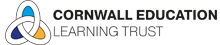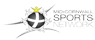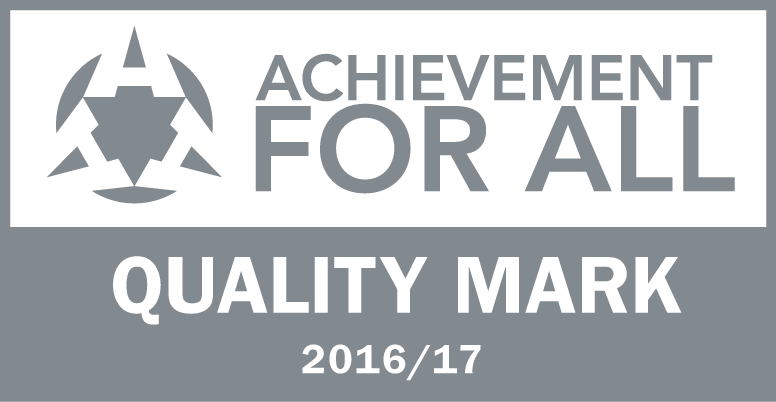# Calculation Methods

This calculation policy is designed to ensure consistency and progression in the teaching of addition, subtraction, multiplication and division across the school.  It is aligned with the 2014 National Curriculum.

Children will use mental calculation approaches as their first port of call when it is efficient and appropriate to do so.  When necessary, an efficient written method needs to be used accurately, confidently and with clear understanding.

Within each section there are examples of concrete (the practical items that pupils can hold and manipulate to help them explore abstract mathematical concepts and the relationships between them), pictorial (models and representations) and abstract (the symbolic stage).

Concrete

Concrete is the “doing” stage, using concrete objects to model problems. Instead of the traditional method of maths teaching, where a teacher demonstrates how to solve a problem, the CPA approach brings concepts to life by allowing children to experience and handle physical objects themselves. Every new abstract concept is learned first with a “concrete” or physical experience.

For example, if a problem is about adding up four baskets of fruit , the children might first handle actual fruit before progressing to handling counters or cubes which are used to represent the fruit.

Pictorial

Pictorial is the “seeing” stage, using representations of the objects to model problems. This stage encourages children to make a mental connection between the physical object and abstract levels of understanding by drawing or looking at pictures, circles, diagrams or models which represent the objects in the problem.

Building or drawing a model makes it easier for children to grasp concepts they traditionally find more difficult, such as fractions, as it helps them visualise the problem and make it more accessible.

Abstract

Abstract is the “symbolic” stage, where children are able to use abstract symbols to model problems (Hauser).

Only once a child has demonstrated that they have a solid understanding of the “concrete” and “pictorial” representations of the problem, can the teacher introduce the more “abstract” concept, such as mathematical symbols. Children are introduced to the concept at a symbolic level, using only numbers, notation, and mathematical symbols, for example +, –, x, ÷ to indicate addition, multiplication, or division.

Although presented as three distinct stages, it would be expected for teaching to go back and forth between each representation to reinforce concepts.

We hope these will help you understand the methods. If you are still unsure, please do see your child's teacher who will be happy to help.

Using a Number Line [End of Y2/Start of Y3]

On a number line with larger hops [Y3]

Starting a more formal vertical method [End of Y3/Start of Y4]

A more formal vertical method (no carrying) [End of Y4/Start of Y5]

A more formal vertical method of addition (with carrying through to the next column) [Y5]

Formal vertical addition (increased carrying) [Y5/Y6]

Subtraction (subtract, take away, minus, greater/less than, more/less than, difference, count back)

Counting back on a number line [End of Y2/Start of Y3]

Counting back on a number line with larger hops [Y3]

Subtraction can also be shown as counting along a number line [Y3]

Beginning a more formal vertical method [End of Y3/Y4]

Adding on, using a vertical method [Y4/Y5]

Formal vertical method (with no carrying) [Y5]

Formal vertical method (made harder by exchanging into the next column) [Y5/Y6]

Formal vertical method using decimals [Y6]

Formal vertical method (exchanging across two columns) [Y6]

Multiplication (multiply, times, multiplied by, product, multiple)

Counting along a number line [End of Y2/Start of Y3]

Partitioning tens and ones [Y3/Y4 (develop by partitioning hundreds, tens and ones)]

Although introduced as a step along the way to develop the understanding of multiplication, the grid method [Y5] is not a recognised method in the new National Curriculum.

VIDEOS TO DEMONSTRATE FORMAL METHOD OF MULTIPLICATION TO BE ADDED
Division (share equally, divide, divided by/into, how many are left, left over, remainder, fraction)

Putting objects into groups [Y2]

Counting on a number line [Y3]

Counting on a number line using multiples of 10 for larger numbers [End of Y3/Y4]

Division is repeated subtraction (using a number line) [End of Y4/Y5]

"Chunking" Method (TO÷O no remainder) [Y5]

"Chunking" method (HTO÷TO with remainders) [End of Y5/Y6]

"Bus Shelter" method [Y6]

Morven Road, Saint Austell, Cornwall, PL25 4PP

0172673911

•••